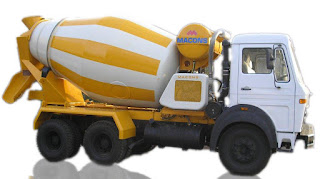The knowledge about "civil engineering" provided by Er. Md Faiz Ali through this plateform is absolutely correct and verified based on practical knowledge and good experiences.

# Quantity of Cement, Sand & AggregateConcrete Mix Design:-
In order to calculate amount of cement, sand and aggregate required in 1m3 of concrete , you have to know about different grades of concrete. Based on strength Different grades of concrete is classified into  M5, M7.5, M10, M15 etc., wheras M stands for Mix and the number behind M stands for characteristic Compressive strength(fck) of the concrete in 28 days when checked in direct compression test. Tthe ratio for M20 grade of concrete is 1:1.5:3 that mean 1 part of cement, 1.5 part of sand (fine aggregate) and 3 parts of aggregate (crushed stone) in volume and then batched for mixing. To know the Concrete Mix Design follow below:-
Mix Design (M) = Cement: Sand :Aggregate

#### Different grades of concrete has different proportions as follows (Concrete Mix Design) :

As Per IS456:2000 the concrete of different grades has following proportions of Cement, sand and aggregate.

 Grade Proportion M5 1:5:10 M7.5 1:4:8 M10 1:3:6 M15 1:2:4 M20 1:1.5:3 M25 1:1:2 M30 1:1:3

#### Calculation of Quantities of Cement, Sand and Aggregate in 1m3 of concrete:-

##### Concrete Mix Design for M20 Grade Concrete:-
Now let us consider M20 grade concrete
As per IS456:2000 M20 Grade concrete proportion is  = 1 : 1.5 : 3
We have to add all the volume to know the total volume = 1 + 1.5 + 3 = 5.5
As we know that  during concreting when we place wet concrete , it gets harden after certain standard time. Considering the same it had be decided upon by Civil design Engineers to take a factor of safety ranging from 1.54 to 1.57 to counter that shrinkage.
i.e volume of dry Concrete = 1.54 to 1.57 times Volume of wet concrete
I am Assuming 1.57 as factor of safety
So total volume of concrete required is 1.57 CumHence we require 8 bags of cement for 1 cum of concrete.
Detailed explanation for No. of bags required for 1 cum of cementSand usually consists of moisture content. It increases the volume of sand (bulking of sand).
For accurate calculation use dry sand or include the effect of bulking in calculation.
Hence we require 672kg of sand  for 1 cu.m of concrete.Density of aggregate may go higher if void spaces decreases. 5mm aggregate has more density when compared with 20mm size of aggregate
Hence We require 1326Kg of 20mm aggregate for 1m3 of Concrete

##### Amount of Water Required for 1m3 of Concrete:-
For making concrete, required amount of water is added. Water quantity is depends upon the climatic factors and workability required. We generally maintain 0.35-0.50 of water cement ratio. Remember, above values  may change according to the moisture content present in sand and aggregate.Hence 200 Litres of Water is required for 1m3 of M20 Concrete
Summary: –
8 bags of cement required for 1 cu.m of M20 grade concrete.
672 kg of Sand required for 1 cu.m of M20 grade concrete.
1326 kg of 20mm Aggregate is required for 1 cu.m of M20 grade concrete.
200 litres of water is required for 1 cu.m of M20 grade concrete